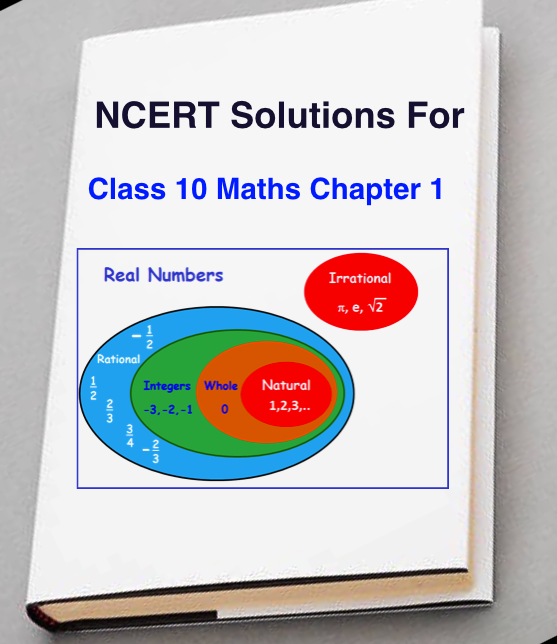# NCERT Solutions for Class 10 Maths Chapter 1

Our Website gives students the best NCERT solutions for class 10 math. we provide for all the learners can have a way to Learn mathematics for Class 10th with the unique solution. Students can download NCERT Solutions for class 10 maths chapter 1 in any time and anywhere. This solution provides you a better and easy understanding way, so students understand the concepts in a very easy and structured way.

Our NCERT solutions for class 10 math are classified into chapters for learners who have difficulty in finding solutions Chapterwise. Here we provided the solution for NCERT Solutions for class 10 maths chapter 1 which is very helpful and can be helpful in your aim of obtaining maximum marks in your board exam.

Not just this, if any student needs more explanation about any concept or topic, they can contact our teachers for individual guidance. you can check  NCERT Solutions For Class 10 Maths Chapter 3 in English MediumStudents Download NCERT Solutions for class 10 maths chapter 1 in Hindi medium based on newest CBSE Syllabus and pattern of 2017 – 18 exams.

The Revision of the book is a very good source of study and it provides a nice way to your preparation and makes your confidence for the exam.

Assignments for each and every chapter, chapter wise exam paper with various difficulty levels, worksheet for practice, full-length practice papers with solution, solved assignments are very useful to study material for the students.

We continue our talk on real numbers in this chapter. We begin with two very important properties of positive integers in Sections 1.2 and 1.3, Euclid’s division algorithm, as the name suggests, has to do with divisibility of integers.

Stated simply, it says any positive integer a can be divided by another positive integer b in such a way that it leaves a remainder r that is smaller than b. Many of you probably recognize this as the usual long division process.

Although this result is quite easy to state and understand, it has many applications related to the divisibility properties of integers. We touch upon a few of them and use it mainly to compute the HCF of two positive integers.

We provide 100% Correct Class Notes of NCERT Solutions for Class 10 Maths Chapter 1 (Real Number) solved by experienced Maths teachers. We give step by step answers to the questions which are in class 10 maths textbook as per CBSE Board instructions and guidelines from the latest NCERT book for class 10 maths. Here a list of all The topics and sub-topics in Chapter 1 Real Number

• 1.1 Introduction,
• 1.2 Euclid’s Division Lemma,
• 1.3 The Fundamental Theorem of Arithmetic,
• 1.4 Revisiting Irrational Numbers,
• 1.5 Revisiting Rational Numbers and Their Decimal Expansions

## NCERT Solutions for Class 10 Maths

EXERCISE 1.1 – Total 5 Questions with Accurate Solutions,

EXERCISE 1.2 – Total 7 Questions with Accurate Solutions,

EXERCISE 1.3 – Total 3 Questions with Accurate Solutions

EXERCISE 1.4 – Total 3 Questions with Accurate Solutions.

ncert-solutions-for-class-10-maths-chapter-1-min

Trending Article:Written by

##### Sonam Sharma

I am govt employ and if you have any question related to govt job then please ping me. Downlaod NCERT Books For Class 10 &NCERT Class 10th Maths Book

This site uses Akismet to reduce spam. Learn how your comment data is processed.